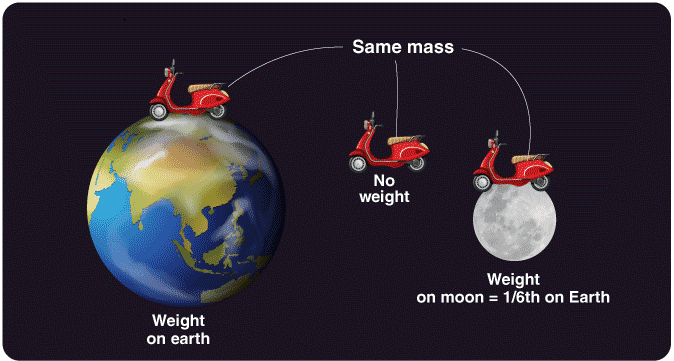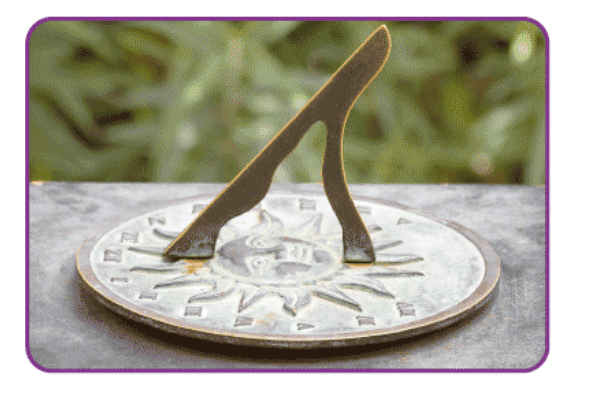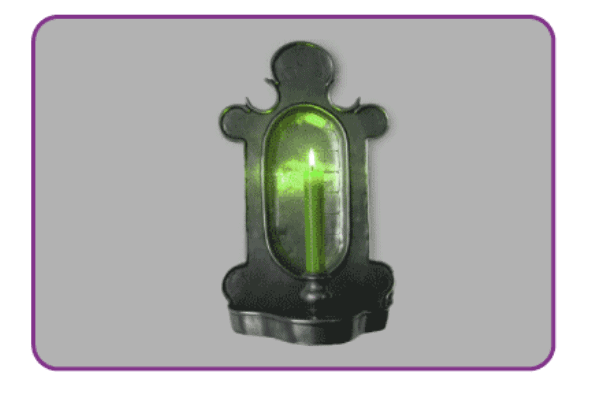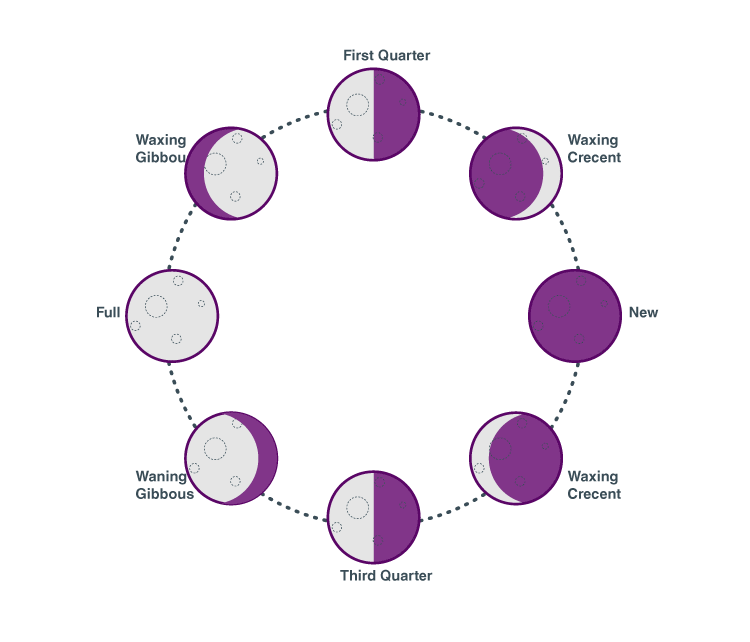Courses

# Measurement of Length, Mass & Time Notes | EduRev

## JEE : Measurement of Length, Mass & Time Notes | EduRev

The document Measurement of Length, Mass & Time Notes | EduRev is a part of the JEE Course Physics For JEE.
All you need of JEE at this link: JEE

Measurements of length and distance are done in many ways. Did you know that you can use the average human body as means to measure? For example, the foot (which is literally the length measurement of an average human foot) is around 25 – 30 cm. This unit of measurement is still in use nowadays. We also use units like inches and yards which are still in use but they are not the standard units of length measurements.

What Is Distance?

Distance is defined as the product of speed and time and it can be represented as follows:
d = s × t
Where,

• d is the distance travelled in m
• t is the time taken to cover the distance in s
• s is the speed in m.s-1

Metric Units of Distance

Most commonly used units of measurement of length are:

• Millimetre
• Centimetre
• Metre
• Kilometre

Following is the table explaining the relationship between all these units:

 Abbreviation Full form Equivalent mm millimetre 10mm = 1cm1000mm = 1m cm centimetre 100cm = 1m m metre 1000m = 1km km kilometre 1000m = 1km

Measurement of Mass: Mass and Weight

Mass is a basic characteristic property of matter. It exists self-sufficiently and is independent of all other parameters such as the temperature, pressure, and the location of the object in space. Atomic mass is the mass of an atom expressed in atomic mass units. The matter has mass and occupies space. These two things are taught to us as soon as we can grasp these concepts. A matter is anything you can touch physically, so everything you see and interact with around you has a mass. Mass is often confused with another parameter. This confusion occurs due to the fact that this parameter is mistakenly used around the globe instead of mass due to its convenience and also due to the fact that we weigh things to find out their mass. This parameter is called weight. Let’s explore both of these essential parameters thoroughly.

What is Mass?

Mass by definition refers to the amount of matter in a particular object. This value of the amount of matter i.e. mass of an object is an intrinsic value of that body and it can help us find out various other parameters that are dependent on the mass. Mass determines the strength of its mutual gravitational attraction to other bodies, its resistance to acceleration due to a force, Inertia, and mass can also be used to derive the energy content of a sample through the theory of Relativity using Albert Einstein’s E = mc2.
The SI Unit of mass is the kilogram (kg). A kilogram can be divided into 1000 grams and it was first defined as one cubic decimetre of water at the melting point of ice, i.e. 0o C. This was a very risky method of defining the unit of mass since the melting point of ice can be altered easily leading to changes in the value of a kilogram. Such changes in the basic units of science can cause a catastrophe which is why the Kilogram was redefined as the mass of the International Prototype Kilogram. International prototype Kilogram is a block of Platinum-Iridium alloy in the shape of a cylinder 39.17 millimetres in both height and diameter. Kilogram serves the function of everyday use perfectly well but it becomes unfeasible as a unit in the case of both extremely large and tiny masses.

Atomic Mass Unit

For tiny and larger objects we use other units;

• Tonne(Metric Ton) is equal to 1000 kg
• The Atomic Mass Unit is used while dealing with atoms and molecules whose masses are so small that the kilogram becomes inconvenient. One atomic mass unit is defined as 1/12th the mass of a Carbon-12 atom. The value of 1 atomic mass unit is obtained as 1.66 x 10-27

Measurement of mass

Measurement of mass is most commonly done by a Balance. The unknown mass of a body is compared with a known value of mass. We obtain the value of an unknown mass in terms of a known value of mass. A balance works in space and in places of no gravity as well since changes in gravity affect both the masses on the balance equally.What is Weight?

Mass is not the same as weight. While mass is the intrinsic property of the body, weight is the measure of the force exerted on the mass of the body due to gravity. Mass refers to a universal value of the object whereas weight is a localized interpretation of the mass of the object. Weight is the effect of gravity and therefore we describe weight with the formula;

W = mg

Where m is the mass and g is the acceleration due to gravity at that particular location. The unit of measurement of weight is Force, the SI Unit of which is Newton. For example, an object that has a mass of 50 kg experiences a gravitational force i.e. weight which is equal to 50 x 9.8 = 490 Newton. So when you tell your friends you weight 50 kg you are telling them about your mass and not your weight. The same object albeit with the same mass of 50 kg will weigh 1/6th on the moon what it did on Earth. Weight and mass mean the same thing on Earth since the effects of gravity are fairly constant throughout the Earth. It was upon our venture into space that it became necessary to create a distinction between mass and weight.Time Measurement By Periodic Events

Evolution of Time Measurement

Time measurement is a complicated task and is always a challenge for us, how do we measure a minute, an hour, a day. Our ancestors noticed that there were some periodic events that never changed, like the changing of seasons, rising and setting of the sun, etc. These were the first attempts mankind had ever made to measure time, it was pretty simple, and the measurements they got were accurate for those times, the first-ever device used to measure time in the daytime was the sundial.Sundial

The oldest sundial was found in Egypt dating back to 3500 BC, a sundial has a dial plate with hour lines engraved on it and a gnomon stick which sticks out at an angle, as the sun moves in the sky it casts a shadow on the plate and shows the time, but it works only in the daytime, so people had to find other ways to measure time at nighttime. A candle clock was used by ancient Chinese to measure time at nighttime; a candle with uniform dimension was used to measure time as the rate at which it would burn for every hour was constant, so markings were made for every inch or so to measure time.Measuring time using a candle

Notice how periodic events like rising and setting of the sun were used for time measurement, Can you say which periodic event should be used to measure a month?

• a) Phases of the moon.
• b) Movement of stars.
• c) Flowing of water.
• d) Changing of seasons.

It takes approximately 29.5 days for the moon to change its phase from one full moon to the next so it’s the closest our ancestors could get to measure a month,Phases of moon

And a year was measured by the changing of seasons, it would take exactly one year for the monsoon to return. So people counted the number of days in one year this way, measurement of a year was very important because we rely on rain for our crops hence knowledge about rain was vital for agriculture. As technology advanced, we devised more and more sophisticated methods to measure time, a simple pendulum was used, as long as the weight of the bob and the length of the pendulum is unchanged, the pendulum would oscillate at a constant rate.Pendulum clock

What mechanism does your wristwatch use for time measurement? Modern clocks make use of a piezoelectric crystal called quartz, you must have read quartz written on a wall clock while checking the time, well this crystal vibrates at a specific frequency when voltage is applied to it, so it is designed in such a way that a frequency of one hertz is obtained from it, this is used to measure time.
In this way periodic events can be used to measure time, here is an assignment for you, what periodic events can you find around your other than the one mentioned in this article to measure time, make a note of them and find a way to measure accurate time using it. This could be helpful for your next science project.

Offer running on EduRev: Apply code STAYHOME200 to get INR 200 off on our premium plan EduRev Infinity!

## Physics For JEE

187 videos|516 docs|263 tests

,

,

,

,

,

,

,

,

,

,

,

,

,

,

,

,

,

,

,

,

,

,

,

,

;